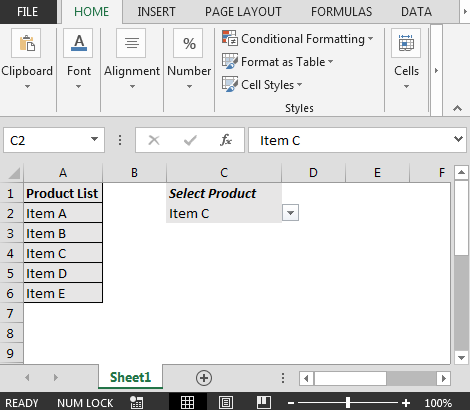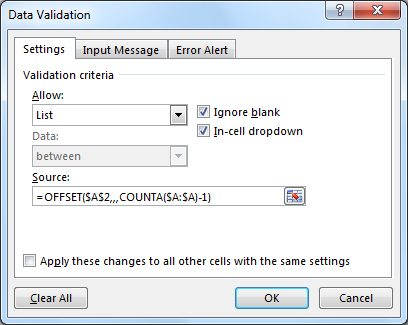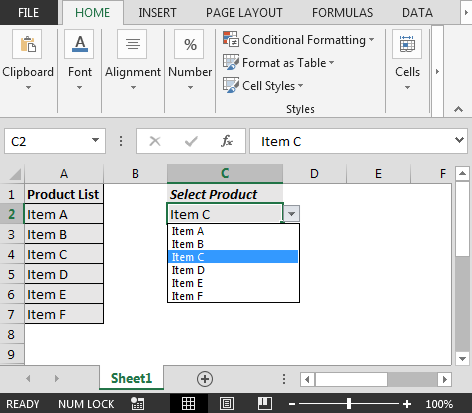# How to Create Dynamic Data Validation Dropdown List in Excel

In Excel, we can limit the user to input values in a cell to a drop down list, using Data Validation. In this article, we will learn constructing a list of dynamic drop down lists in Excel.

Let's take an example to understand:-

Question: I know how to create data validation in Excel. I mean static dropdown list while I want a quick way in Excel to create a data validation list based on the values enter & immediately the validation list which is linked with the input values to be populated in preset data validation list.

The function used in this tutorial will work on following versions of Microsoft Excel:

Excel 2013, Excel 2010, Excel 2007, Excel 2003

Following is the snapshot of Static dropdown list:Let us follow the below steps to create dynamic dropdown list in Excel.

• We can use OFFSET function to make dynamic data validation list
• Press ALT + D + L
• From Settings tab; click on Allow
• In Source box, enter the following formula
• =OFFSET(\$A\$2,,,COUNTA(\$A:\$A)-1)• Click on ok button & dynamic data validation is ready for use in Excel.In this way, we can anytime add to our existing data validation list & remove data validation in Excel.